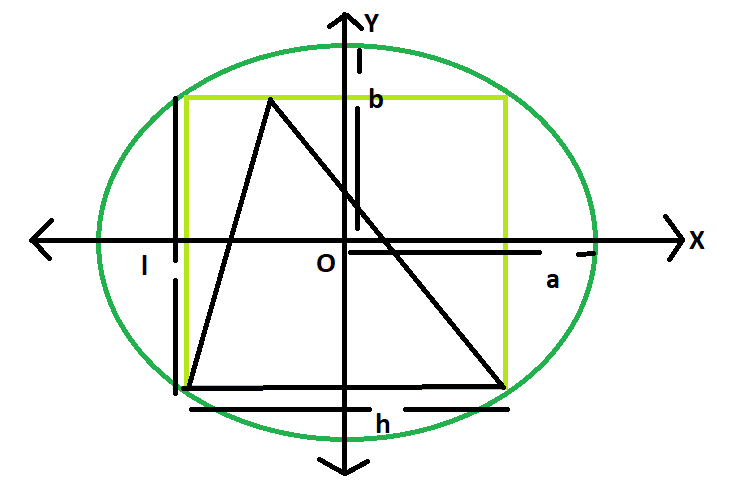# Area of a triangle inscribed in a rectangle which is inscribed in an ellipse

Given here is an ellipse with axes length 2a and 2b, which inscribes a rectangle of length l and breadth h, which in turn inscribes a triangle.The task is to find the area of this triangle.

Examples:

```Input: a = 4, b = 3
Output: 12

Input: a = 5, b = 2
Output: 10
```

## Recommended: Please try your approach on {IDE} first, before moving on to the solution.Approach:
We know the Area of the rectangle inscribed within the ellipse is, Ar = 2ab(Please refer here),
also the area of the triangle inscribed within the rectangle s, A = Ar/2 = ab(Please refer here)

Below is the implementation of the above approach:

## C++

 `// C++ Program to find the area of the triangle ` `// inscribed within the rectangle which in turn ` `// is inscribed in an ellipse ` `#include ` `using` `namespace` `std; ` ` `  `// Function to find the area of the triangle ` `float` `area(``float` `a, ``float` `b) ` `{ ` ` `  `    ``// length of a and b cannot be negative ` `    ``if` `(a < 0 || b < 0) ` `        ``return` `-1; ` ` `  `    ``// area of the triangle ` `    ``float` `A = a * b; ` `    ``return` `A; ` `} ` ` `  `// Driver code ` `int` `main() ` `{ ` `    ``float` `a = 5, b = 2; ` `    ``cout << area(a, b) << endl; ` `    ``return` `0; ` `} `

## Java

 `   ``// Java Program to find the area of the triangle ` `// inscribed within the rectangle which in turn ` `// is inscribed in an ellipse ` ` `  `import` `java.io.*; ` ` `  `class` `GFG { ` ` `  `// Function to find the area of the triangle ` `static` `float` `area(``float` `a, ``float` `b) ` `{ ` ` `  `    ``// length of a and b cannot be negative ` `    ``if` `(a < ``0` `|| b < ``0``) ` `        ``return` `-``1``; ` ` `  `    ``// area of the triangle ` `    ``float` `A = a * b; ` `    ``return` `A; ` `} ` ` `  `// Driver code ` ` `  ` `  `    ``public` `static` `void` `main (String[] args) { ` `    ``float` `a = ``5``, b = ``2``; ` `    ``System.out.println(area(a, b)); ` `    ``} ` `} ` `//This code is contributed by anuj_67.. `

## Python3

 `# Python 3 Program to find the  ` `# area of the triangle inscribed  ` `# within the rectangle which in  ` `# turn is inscribed in an ellipse ` ` `  `# Function to find the area ` `# of the triangle ` `def` `area(a, b): ` `     `  `    ``# length of a and b cannot  ` `    ``# be negative ` `    ``if` `(a < ``0` `or` `b < ``0``): ` `        ``return` `-``1` ` `  `    ``# area of the triangle ` `    ``A ``=` `a ``*` `b ` `    ``return` `A ` ` `  `# Driver code ` `if` `__name__ ``=``=` `'__main__'``: ` `    ``a ``=` `5` `    ``b ``=` `2` `    ``print``(area(a, b)) ` `     `  `# This code is contributed  ` `# by Surendra_Gangwar `

## C#

 `// C# Program to find the area of  ` `// the triangle inscribed within  ` `// the rectangle which in turn ` `// is inscribed in an ellipse ` `using` `System; ` ` `  `class` `GFG ` `{ ` `     `  `// Function to find the  ` `// area of the triangle ` `static` `float` `area(``float` `a, ``float` `b) ` `{ ` ` `  `    ``// length of a and b  ` `    ``// cannot be negative ` `    ``if` `(a < 0 || b < 0) ` `        ``return` `-1; ` ` `  `    ``// area of the triangle ` `    ``float` `A = a * b; ` `    ``return` `A; ` `} ` ` `  `// Driver code ` `static` `public` `void` `Main () ` `{ ` `    ``float` `a = 5, b = 2; ` `    ``Console.WriteLine(area(a, b)); ` `} ` `} ` ` `  `// This code is contributed by ajit `

## PHP

 ` `

Output:

```10
```

Attention reader! Don’t stop learning now. Get hold of all the important DSA concepts with the DSA Self Paced Course at a student-friendly price and become industry ready.

My Personal Notes arrow_drop_upCheck out this Author's contributed articles.

If you like GeeksforGeeks and would like to contribute, you can also write an article using contribute.geeksforgeeks.org or mail your article to contribute@geeksforgeeks.org. See your article appearing on the GeeksforGeeks main page and help other Geeks.

Please Improve this article if you find anything incorrect by clicking on the "Improve Article" button below.